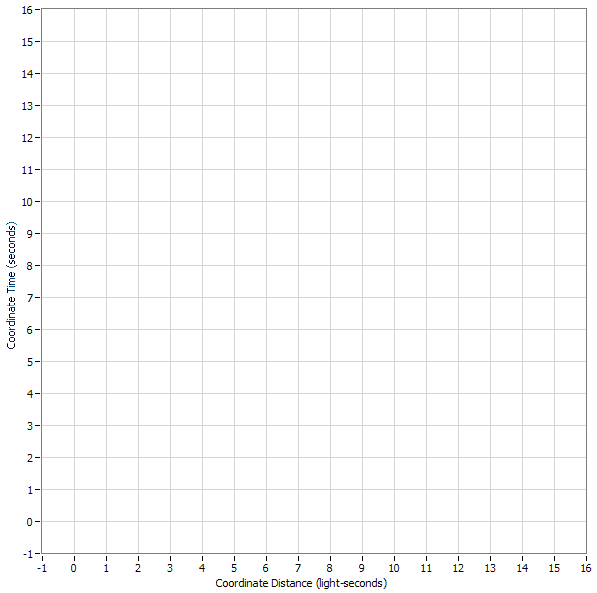# Spacetime diagram drawing problem

• Trojan666ru
In summary, the object moving away from me with a velocity of 50% speed of light will be "half light second away" at the same time t=1 in my clock.f

#### Trojan666ru

I was drawing a spacetime diagram to relate Doppler shift effect but i stuck at a point which i can't understand
This is what I'm trying to draw
An object with mirror is moving away from me with a velocity of 50% speed of light
When each second passes in my clock i send light pulses at that object.
I sent 4 light pulses in 4 second.
The object starts when t=0 in my clock
When t=1 in my clock that object will be ’half light second away’ at the same time t=1 i sent my first light pulse. So in my clock the first light pulse must hit the object when t=2 in my clock (when t=2 in my clock the object will be 1 light second away)
When t=2 i sent my 2nd light pulse and it must hit the object when t=4 in my clock
When t=3 i sends my 3rd light pulse & it must hit the object when t=6 in my clock
Finally t=4 i sends my 4th light pulse and it must hit the object when t=8 in my clock
So i draw my spacetime diagram and drew a 22.5 degree angle, it will be 50% speed of light
In each seconds i sends a light pulse, so i drew it in 45 degree angle.
But the intersection of light pulse and speed of the object doesn't match with time in my graph
The first light pulse which i sent should intersect at t=2 in my graph but it intersect only below 2 second.
So if i try to adjust the graph inorder to reach at t=2 the angle changes and it will increase the speed of the object. This is like the same for all 4 light pulses
I know moving objects contract in length, but this isn't that
I also know time slows in moving object, but i don't need to care, i need my spacetime graph to be correct, they may receive it in different times
If it is my mistake help me to find it.
The picture is below

http://www.flickr.com/photos/sreenath666/10286909563/ [Broken]

Last edited by a moderator:
Try using an Arctan(1/2) = 26.6 degrees angle.

That will change the speed of the object

Oops, I meant 45 degrees - Arctan(1/2) = 18.4 degrees.

anyway changing angle will increase or decrease its speed, i want it going at 50% speed of light

Then you need Arctan(1/2) between the ct-axis and the ct'-axis, and thus 45 degrees -Arctan(1/2) between the ct'-axis and the light diagonal.

"50% of the speed of light" is a line with slope (1/2) on an x-vs-t graph.
Use your grid boxes to determine that slope...
for every (say) 4 seconds, the mirror has traveled another 2 light-seconds.
Don't use a [Euclidean] protractor on a Minkowski spacetime diagram.

by the way, do someone studying for pHd draw 50% in ct x diagram like u say?
They draw it exact at the half position, and if it is wrong in using Euclidean tools, then isn't it wrong to draw a 45 degree line in spacetime diagram? we still use protractor for that

To get a slope 1/2 between the horizontal and a line, you do *not* bisect the 45 degrees angle. Instead you draw a line with slope 1/2, which means climbing 1 unit for every 2 units of horizontal displacement. That gives an angle of 26.6 degrees, not one of 22.5 degrees.
See https://en.wikipedia.org/wiki/Slope

Last edited:
You can use a protractor to draw a 45-degree line...
or you can use your grid, which helps you avoid having to use any special tools.
(It's not wrong to use a protractor...
but it is tedious [compared to counting boxes in a grid]
and it is unnatural since the geometry of a spactime diagram [or even a Galilean spacetime diagram [i.e, the ordinary phy 101 x-vs-t graph] ] is not Euclidean.)

Note that the relation between slope v and Euclidean-angle is
v=tan(theta), which implies that v and theta do not scale proportionally.
Doubling theta does not result in doubling v.

(Using rapidity in Minkowskian geometry, v=tanh(rapidity), which also implies that v and rapidity do not scale proportionally.)